# AP Statistics : How to identify independent events

## Example Questions

← Previous 1

### Example Question #1 : How To Identify Independent Events

Given a pair of fair dice, what is the probability of rolling a 7 in one throw?

1/3

5/6

1/6

1/4

1/2

1/6

Explanation:

There are 36 total outcomes for this experiment and there are six ways to roll a 7 with two dice: 1,6; 6,1; 2,5; 5,2; 3,4; and 4,3. Thus, 6/36 = 1/6.

### Example Question #2 : How To Identify Independent Events

A fair coin is tossed into the air a total of ten times and the result, heads or tails, is the face landing up. What is the total number of possible outcomes for this experiment?

2

20

512

100

1024

1024

Explanation:

There are two outcomes in each trial of this experiment, and there are ten total trials. Thus, 2 raised to the tenth power yields an answer of 1024.

### Example Question #3 : How To Identify Independent Events

Mary randomly selects the king of hearts from a deck of cards.  She then replaces the card and again selects one card from the deck.  The selection of the second card is a(n)_________ event.

dependent

independent

independent

Explanation:

The selection of the second card is an independent event because it is unaffected by the first event.  If the king of hearts had not been replaced, then the probability of selecting a particular card would have been affected by the first event, and the second selection would have been dependent.  This, however, is not the case in this question.

### Example Question #1 : Understanding Quartiles And Percentiles

What is the probability of getting a sum of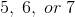when rolling two six-sided fair dice?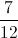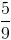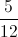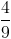Explanation:

The sample space, or total possible outcomes, when rolling two six-sided dice is.

Ways to get what you want: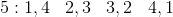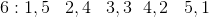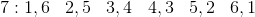So there areways to get a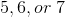.

So the probability becomes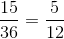### Example Question #1 : Independent And Dependent Events

Each answer choice describes two events.  Which of the following describes independent events?

A coin is flipped in the air and the result is heads.  The coin is flipped in the air again.

A marble is randomly drawn from a bag and set aside. A second marble is drawn from the bag.

A card is drawn from a pile and set aside.  A second card is drawn.

One of ten differently colored candies is selected from a jar and eaten.  A second candy is then randomly selected.

A shirt is randomly selected from a drawer and is placed in the laundry.  A second shirt is randomly slected from the drawer.

A coin is flipped in the air and the result is heads.  The coin is flipped in the air again.

Explanation:

Two events are independent of each other when the result of one does not affect the result of the other.  In the case of the coin being flipped, the first result in no way influenced the result of the second coin flip.  In contrast, when a card is removed from a deck of cards and set aside, that card cannot be selected when a second card is taken from the deck.

### Example Question #1 : How To Identify Independent Events

Eventsandare known to be independent.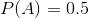while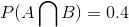. What must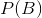be?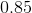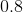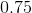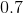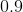Explanation:

Because the two events are known to be independent, then the following is true by definition.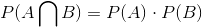.

This then becomes an algebra problem: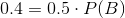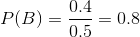### Example Question #1 : Independent And Dependent Events

Two eventsandare independent, and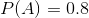while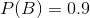. What is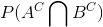?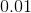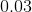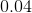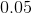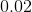Explanation:

Because the two are independent, the calculation becomes the product of the two by definition.

We need to recall thatrespresents the compliment of A which is everything that is not in A or in mathematical terms: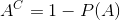.

Likewise for the compliment of B: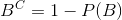Therefore to find the intersection of these two independent events we multiply them together.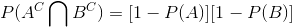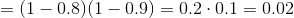### Example Question #7 : How To Identify Independent Events

True or false:

A family has 3 boys. The probability that the fourth child will also be a boy is less than 50%

Sometimes

False

True

False

Explanation:

The gender of each child can be considered an independent event. Each child has a 50% chance of being a boy, and whether a boy was already born previously does not affect the next child's gender.

### Example Question #8 : How To Identify Independent Events

True or false: When drawing two cards with replacement, the event drawing a spade first is independent of the event drawing a heart second.

True

False

Sometimes

True

Explanation:

These two events are independent of one another. During sampling with replacement, the first card does not affect the second card being picked.

To illustrate, consider the probability of drawing a heart first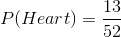Assuming you first drew a heart and replaced it in the deck, does the probability of drawing a heart as the second card change?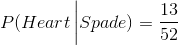The probability remains the same, there are still 13 hearts and 52 total cards.

### Example Question #9 : How To Identify Independent Events

True or False: When 2 cards are drawn without replacement from a regular deck of 52 cards, the event of drawing a heart first independent of the event of drawing a heart second.

True

False

Sometimes

Cannot be determined by the information given.

False

Explanation:

These events are not independent, because if one event happens, it affects the probability of the other event happening. Consider the probability of drawing a heart and the probability of getting a heart given a heart was already drawn. If these two probabilities are the same, the events are independent. If the two probabilities are not the asme, the events are not independent.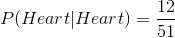After a heart has already been drawn, there are now only 52 cards total and 12 hearts left. These two probabilities are not equal, therefore the events are not independent.

← Previous 1